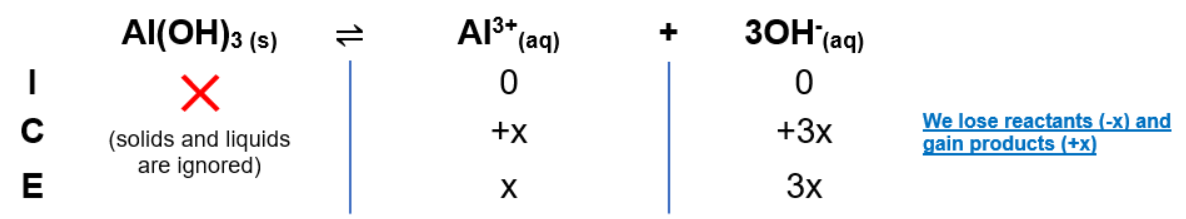# Problem: Enter the solubility-product expression for Al(OH)3(s).

###### FREE Expert Solution

Recall that Ksp is an equilibrium constant related to slightly soluble ionic compounds

The dissociation of slightly soluble Al(OH)3 in solution is as follows:

Al(OH)3(s)   Al3+(aq) + 3 OH(aq)

We can construct an ICE table for this dissociationwhere x = molar solubility of the compound.

The Ksp expression is:

$\overline{){{\mathbf{K}}}_{{\mathbf{sp}}}{\mathbf{=}}\frac{\mathbf{products}}{\overline{)\mathbf{reactants}}}{\mathbf{=}}\mathbf{\left[}{\mathbf{Al}}^{\mathbf{3}\mathbf{+}}\mathbf{\right]}\mathbf{\left[}{\mathbf{OH}}^{\mathbf{-}}\mathbf{\right]}}$

Remember that solids are ignored in the ICE table and Ksp expression.

97% (118 ratings)###### Problem Details

Enter the solubility-product expression for Al(OH)3(s).